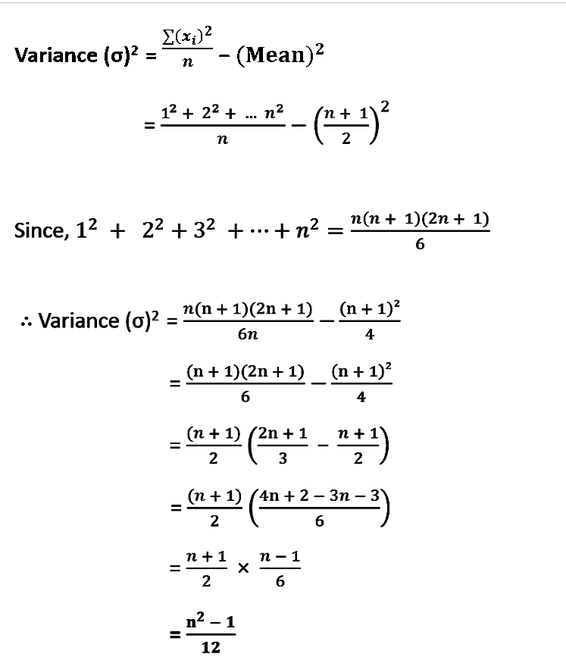# Program to calculate Variance of first N Natural Numbers

• Difficulty Level : Easy
• Last Updated : 02 Sep, 2021

Given an integer N, the task is to find the variance of the first N natural numbers.

Variance is used to determine how far the data is spread from their average value. It is generally represented by symbol σ2 and the equation for finding the variance is generally given by the following equation:

Ni = 1 (xi– mean(x))2 / N

where N is the total number of data

Examples:

Input: 5
Output: 2
Explanation:
Mean of the first 5 numbers = (1 + 2 + 3 + 4 + 5) / 5 = 3
Therefore, Variance = ((1 – 3)2 + (2 – 3)2 + (3 – 3)2+(4 – 3)2+(5 – 3)2) / 5 = (4 + 1 + 0 + 1 + 4) / 5 = 10 / 5.

Input: 4
Output: 1.25
Explanation:
Mean of first 4 numbers = (1 + 2 + 3 + 4) / 4 = 2.5
Therefore, Variance = ((1 – 2.5)2 + (2 – 2.5)2 + (3 – 2.5)2 + (4 – 2.5)2) / 4 = (2.25 + 0.25 + 0.25 + 2.25) / 4 = 5 / 4.

Naive approach: The simple approach to solve this problem is to first calculate the Mean value of the first N natural numbers and then, traverse over the range [1, N] and calculate the variance.

Time Complexity: O(N)
Auxiliary Space: O(1)

Efficient solution: The above solution can be optimized by simplifying the above-mentioned formulas of Mean and Variance and using the properties of sum of the first N natural number and the sum of the squares of the first N natural numbers, as shown below..Therefore, calculate (N2 – 1) / 12 and print it as the required result.

Below is the implementation of the above approach:

## C++

 `// C++ Program to implement` `// the above approach`   `#include ` `using` `namespace` `std;`   `// Function to calculate Variance` `// of first N natural numbers` `long` `double` `find_Variance(``int` `n)` `{` `    ``long` `long` `int` `numerator = n * n - 1;` `    ``long` `double` `ans = (numerator * 1.0) / 12;` `    ``return` `ans;` `}`   `// Driver Code` `int` `main()` `{` `    ``int` `N = 5;`   `    ``cout << fixed << setprecision(6)` `         ``<< find_Variance(N);` `}`

## Java

 `// Java program to implement` `// the above approach` `class` `GFG{`   `// Function to calculate Variance` `// of first N natural numbers` `static` `double` `find_Variance(``int` `n)` `{` `    ``long`  `numerator = n * n - ``1``;` `    ``double` `ans = (numerator * ``1.0``) / ``12``;` `    ``return` `ans;` `}`   `// Driver Code` `public` `static` `void` `main(String[] args)` `{` `    ``int` `N = ``5``;` `    `  `    ``System.out.println(find_Variance(N));` `}` `}`   `// This code is contributed by AnkThon`

## Python3

 `# Python3 program to implement` `# the above approach`   `# Function to calculate Variance` `# of first N natural numbers` `def` `find_Variance(n):` `    `  `    ``numerator ``=` `n ``*` `n ``-` `1` `    ``ans ``=` `(numerator ``*` `1.0``) ``/` `12` `    `  `    ``return` `ans`   `# Driver Code` `if` `__name__ ``=``=` `'__main__'``:` `    `  `    ``N ``=` `5`   `    ``a ``=` `find_Variance(N)`   `    ``print``(``"{0:.6f}"``.``format``(a)) `   `# This code is contributed by mohit kumar 29`

## C#

 `// C# program to implement` `// the above approach` `using` `System;` `class` `GFG` `{`   `    ``// Function to calculate Variance` `    ``// of first N natural numbers` `    ``static` `double` `find_Variance(``int` `n)` `    ``{` `        ``long`  `numerator = n * n - 1;` `        ``double` `ans = (numerator * 1.0) / 12;` `        ``return` `ans;` `    ``}` `    `  `    ``// Driver Code` `    ``public` `static` `void` `Main(``string``[] args)` `    ``{` `        ``int` `N = 5;` `        ``Console.WriteLine(find_Variance(N));` `    ``}` `}`   `// This code is contributed by AnkThon`

## Javascript

 ``

Output:

`2.000000`

Time Complexity: O(1)
Auxiliary Space: O(1)

My Personal Notes arrow_drop_up
Recommended Articles
Page :